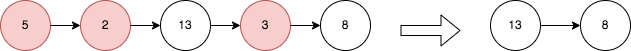# 2487. Remove Nodes From Linked List

Medium
You are given the `head` of a linked list.
Remove every node which has a node with a strictly greater value anywhere to the right side of it.
Return the `head` of the modified linked list.
Example 1:Output:
[13,8]
Explanation:
The nodes that should be removed are 5, 2 and 3.
- Node 13 is to the right of node 5.
- Node 13 is to the right of node 2.
- Node 8 is to the right of node 3.
Example 2:
Output:
[1,1,1,1]
Explanation:
Every node has value 1, so no nodes are removed.
Constraints:
• The number of the nodes in the given list is in the range `[1, 105]`.
• `1 <= Node.val <= 10^5`

### 解題

/**
* type ListNode struct {
* Val int
* Next *ListNode
* }
*/
stack := make([]*ListNode, 0)
for len(stack) != 0 {
top := stack[len(stack) - 1]